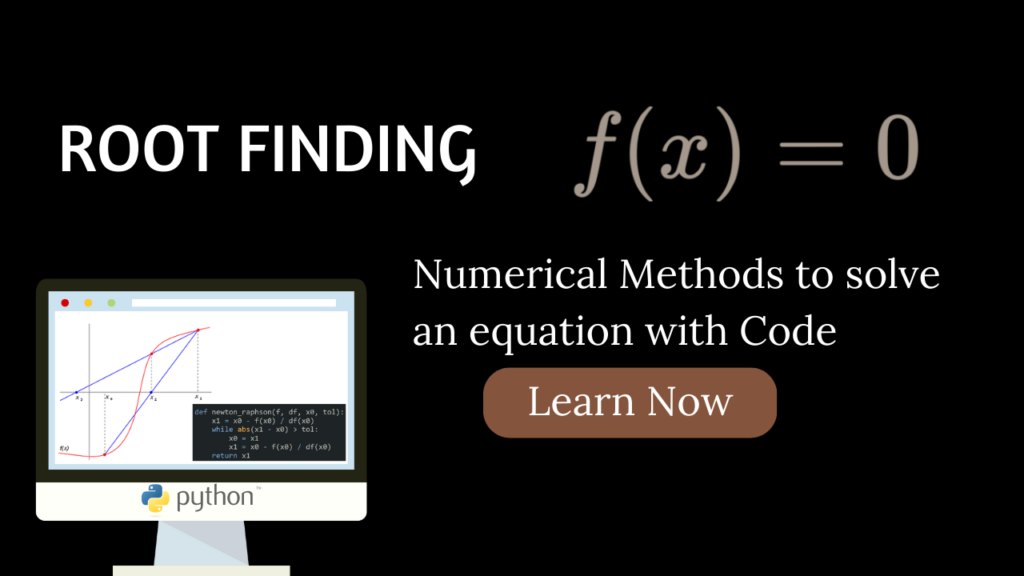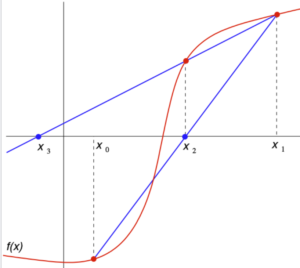# Numerical Methods for Root Finding – with Python codeFinding the roots of an equation, also known as solving for the zeroes, is a fundamental problem in mathematics. There are many different numerical methods that can be used to find the roots of an equation, each with its own advantages and disadvantages. In this blog post, I will discuss five of the most commonly used methods: bisection, secant, Newton-Raphson, false position, and fixed-point iteration. I will also provide Python code snippets and examples for each method, as well as discuss the pros and cons of each method. For all the methods I will try to find the root of the following equation:$x^3-x^2+2 = 0$

The above polynomial has three roots, out of which only one is real, which is -1.0. Therefore, for all the algorithms that we discuss in this blog-post this is the desired answer.

Let’s begin our python program by defining it and also importing some useful modules that we may require later on:

from math import *
import numpy as np

def f(x):
return x**3 - x**2 + 2


### Bisection Method

The bisection method is one of the most basic and widely used methods for finding the roots of an equation. The basic idea behind the bisection method is to repeatedly bisect an interval and then select a subinterval in which a root must lie for further processing. The process is then repeated on the subinterval.

The bisection method can be represented mathematically as:$x_{n+1} = \frac{a_n + b_n}{2}$

Where$x_{n+1}$ is the midpoint of the interval$[a_n, b_n]$ and$f(x_{n+1})$ is the function evaluated at the midpoint.

Here is a Python code snippet for the bisection method:

def bisect(f, a, b, tol):
if f(a) * f(b) >= 0:
print("You have not assumed right a and b. They should bracket the root.\n")
return
c = a
while (b-a) >= tol:
c = (a+b)/2
if f(c) == 0.0:
break
elif f(c)*f(a) < 0:
b = c
else:
a = c
return c



Here is an example of using the bisection method to find the root of the equation$x^3 - x^2 + 2=0$:

root = bisect(f, -4, 0, 0.0001)
print("The root is: ", root)


The output of this code will be:

The root is:  -1.0


The bisection method is a relatively simple and robust method for finding roots of an equation, but it can be slow for some equations and may not converge if the function is not continuous.

#### Related:

A while back I also created a visual animation of the bisection method in action, that maybe of interest to you:

The code used to create the animation can be found here.

### Secant Method

The secant method is similar to the bisection method, but instead of bisecting the interval at each step, the secant method uses the slope of the secant line to approximate the root.

The secant method can be represented mathematically as:$x_{n+1} = x_n - \frac{f(x_n)(x_n - x_{n-1})}{f(x_n) - f(x_{n-1})}$

Where$x_{n+1}$ is the root approximation,$x_n$ and$x_{n-1}$ are the previous approximations, and$f(x_n)$ and$f(x_{n-1})$ are the function evaluated at those approximations.The first two iterations of the secant method. The red curve shows the function f, and the blue lines are the secants. For this particular case, the secant method will not converge to the visible root.Source: https://en.wikipedia.org/wiki/Secant_method#/media/File:Secant_method.svg

Here is a Python code snippet for the secant method:

def secant(f, x0, x1, tol):
x2 = x1 - (f(x1) * (x1 - x0)) / (f(x1) - f(x0))
while abs(x2 - x1) > tol:
x0 = x1
x1 = x2
x2 = x1 - (f(x1) * (x1 - x0)) / (f(x1) - f(x0))
return x2


Here is an example of using the secant method to find the root of the equation$x^3 - x^2 + 2=0$:

root = secant(f, -4, 1, 0.0001)
print("The root is: ", root)


The output of this code will be:

The root is:  -0.9999999998113451


The secant method is generally faster than the bisection method, but it can be less reliable if the initial approximations are not close to the root.

### Newton-Raphson Method

The Newton-Raphson method is a more sophisticated method that uses the derivative of the function to approximate the root. The basic idea behind the Newton-Raphson method is that if we have a good approximation for the root, then the tangent line at that point will be a good approximation for the function near the root.

The Newton-Raphson method can be represented mathematically as:$x_{n+1} = x_n - \frac{f(x_n)}{f'(x_n)}$

Where$x_{n+1}$ is the root approximation,$x_n$ is the previous approximation,$f(x_n)$ is the function evaluated at the approximation, and$f'(x_n)$ is the derivative of the function evaluated at the approximation.

Here is a Python code snippet for the Newton-Raphson method:

def newton_raphson(f, df, x0, tol):
x1 = x0 - f(x0) / df(x0)
while abs(x1 - x0) > tol:
x0 = x1
x1 = x0 - f(x0) / df(x0)
return x1


Here is an example of using the Newton-Raphson method to find the root of the equation$x^3 - x^2 + 2=0$, that we have defined earlier. Note, this time we also need to define its derivative.

def df(x):
return 3*x**2 - 2*x

root = newton_raphson(f, df, 1, 0.0001)
print("The root is: ", root)


The output of this code will be:

The root is:  -1.0


The Newton-Raphson method is generally faster and more accurate than the bisection and secant methods, but it can be less reliable if the initial approximation is not close to the root or if the function is not well-behaved (i.e., it has multiple roots or a derivative that is not continuous).

### False Position Method (Regula Falsi)

The False Position method is an extension of the bisection method that uses a secant line to approximate the root. The basic idea behind the False Position method is that instead of bisecting the interval at each step, we use the secant line that passes through the two endpoints of the interval to approximate the root.

The False Position method can be represented mathematically as:$x_{n+1} = a_n + \frac{f(a_n)(b_n - a_n)}{f(b_n) - f(a_n)}$

Where$x_{n+1}$ is the root approximation,$a_n$ and$b_n$ are the endpoints of the interval, and$f(a_n)$ and$f(b_n)$ are the function evaluated at those endpoints.

Here is a Python code snippet for the False Position method:

def false_position(f, a, b, tol):
if f(a) * f(b) >= 0:
print("You have not assumed right a and b. They should bracket the root.\n")
return
c = a
while abs(f(c)) >= tol:
c = b - f(b)*(b-a)/(f(b)-f(a))
if f(c) == 0.0:
break
elif f(c)*f(a) < 0:
b = c
else:
a = c
return c


Here is an example of using the False Position method to find the root of the equation$x^3 - x^2 + 2=0$:

root = false_position(f, -4, 0, 0.0001)
print("The root is: ", root)


The output of this code will be:

The root is:  -0.999981285497747


The False Position method is generally faster than the bisection method and it can be more reliable in some cases, but it can be less reliable if the function is not well-behaved.

### Fixed-Point Iteration

The Fixed-Point Iteration method is a method that is used to find the roots of an equation of the form $f(x) = x$. The basic idea behind the Fixed-Point Iteration method is to iterate on a function $g(x)$ such that the root of $f(x) = x$ is the fixed point of $g(x)$.

The Fixed-Point Iteration method can be represented mathematically as:$x_{n+1} = g(x_n)$

Where$x_{n+1}$ is the root approximation,$x_n$ is the previous approximation and$g(x_n)$ is the function that will be iterated.

Here is a Python code snippet for the Fixed-Point Iteration method:

def fixed_point_iteration(g, x0, tol):
x1 = g(x0)
while abs(x1 - x0) > tol:
x0 = x1
x1 = g(x0)
return x1


Here is an example of using the Fixed-Point Iteration method to find the root of the equation$x^3 - x^2 + 2 = 0$:

def g1(x):
return -2/(x**2-x)
root = fixed_point_iteration(g1, -4, 0.0001)
print("The root is: ", root)


The output of this code will be:

OverflowError: (34, 'Result too large')


This is because the iterative process has gone into an infinite loop without converging the value of$x1$ is tending to infinity. Even changing the initial guess doesn’t work.

Let’s try a different function now:

def g2(x):
return np.cbrt(-2 + x**2)
root = fixed_point_iteration(g2, -4, 0.0001)
print("The root is: ", root)


The output of this code will be:

The root is:  -0.9999725506219916


As seen above, the fixed-point iterative method can be a bit troublesome for some definitions of$g(x)$.

In conclusion, there are many different numerical methods that can be used to find the roots of an equation, each with its own advantages and disadvantages. The bisection method is simple and robust but slow, secant method is faster than bisection but less reliable, Newton-Raphson method is faster and more accurate but can be less reliable if the initial approximation is not close to the root or if the function is not well-behaved, False Position method is generally faster than bisection and can be more reliable in some cases, Fixed-Point Iteration method is generally faster but also dependent on the definition of$g(x)$.

### References:

Note: The above code snippets are just examples and should be used with care and proper testing before use in any real-world scenario.

[wpedon id="7041" align="center"]

This site uses Akismet to reduce spam. Learn how your comment data is processed.